# 算法与数据结构（3）：基本数据结构——链表，栈，队列，有根树

2019/04/10 10:10

### 链表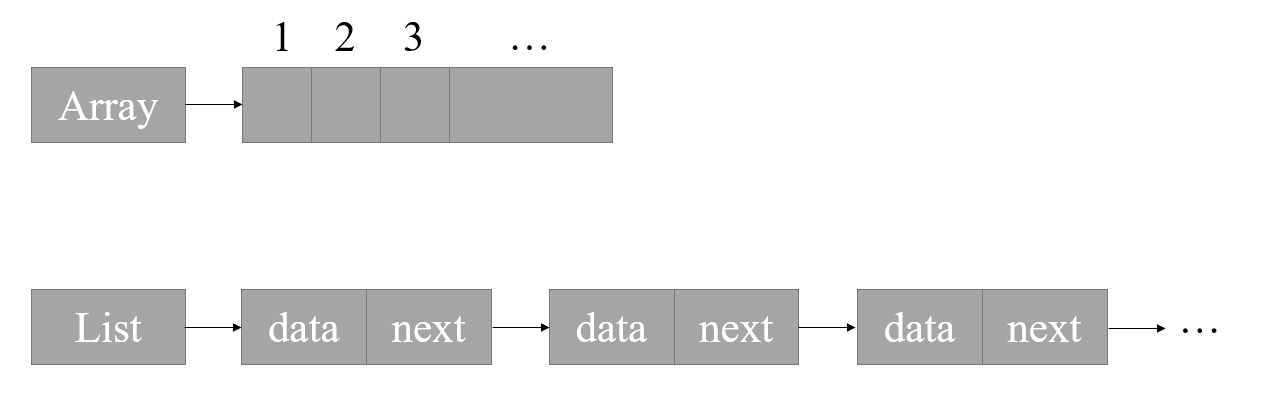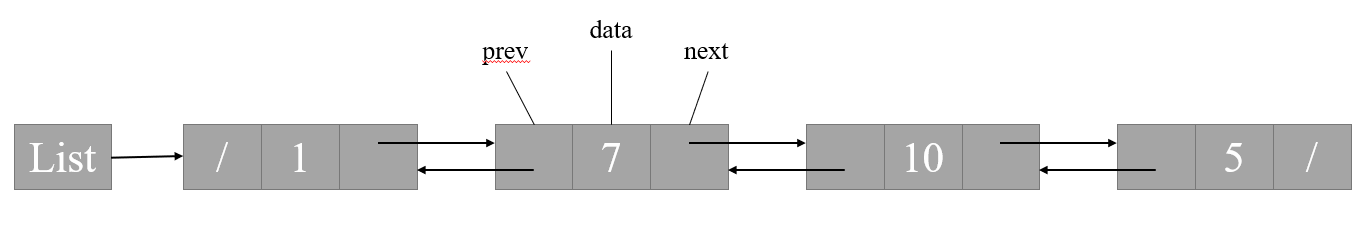// 列表节点
typedef struct ListNode{
int data;
struct ListNode* prev;
struct ListNode* next;
}ListNode;


// 列表
typedef struct List{
int length;
}List;


// 初始化一个列表，不包含任何数据
List* ListInit(){
List* list = (List*)malloc(sizeof(List));
list->length = 0;
return list;
}


// 在list列表中搜索data为number_to_search的第一个节点，并返回此节点
ListNode* ListSearch(List* list, int number_to_search){
while(node != NULL && node->data != number_to_search){
node = node->next;
}
return node;
}


// 新建一个data为number_to_add的节点，并将其添加至list列表头部
// 新建节点

// 将新节点插入至列表头部
}
list->length ++;
return 0;
}


// 从list列表中删除node节点，并free掉node节点
int ListDelete(List* list, ListNode* node){
if(node->prev == NULL){
} else{
node->prev->next = node->next;
}

if(node->next != NULL){
node->next->prev = node->prev;
}
free(node);
list->length --;

return  0;
}


### 栈和队列

#### 栈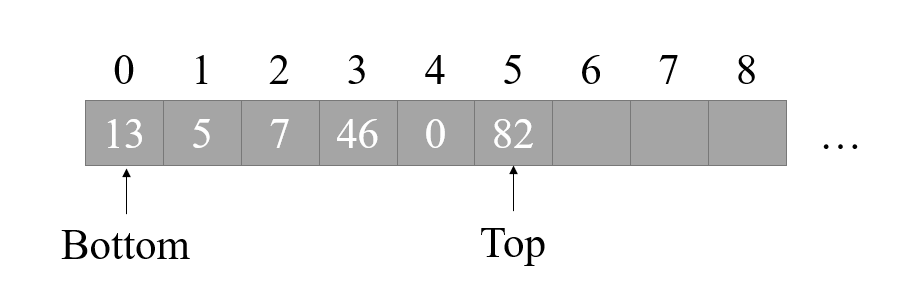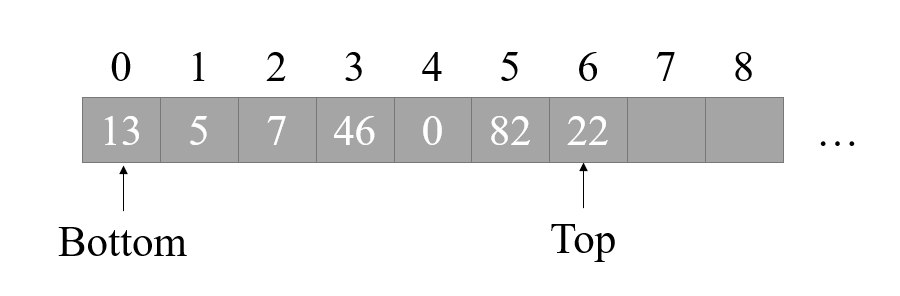// 定义栈
typedef struct Stack{
int* array;
int top;
int length;
}Stack;


// 初始化栈
Stack* StackInit(int stack_length){
Stack* stack = (Stack*)malloc(sizeof(Stack));
stack->array = (int*)malloc(sizeof(int) * stack_length);
stack->top = -1;
stack->length = stack_length;
return stack;
}


// 判断栈是否已满
int StackIsFull(Stack* stack){
return (stack->top >= (stack->length - 1));
}

// 判断栈是否为空
int StackIsEmpty(Stack* stack){
return (stack->top < 0);
}


// 压栈
int StackPush(Stack* stack, int number_to_push){
if(StackIsFull(stack)){
return -1;
} else{
stack->top ++;
stack->array[stack->top] = number_to_push;
return 0;
}
}


// 出栈
int StackPop(Stack* stack){
stack->top --;
return stack->array[stack->top + 1];
}


#### 队列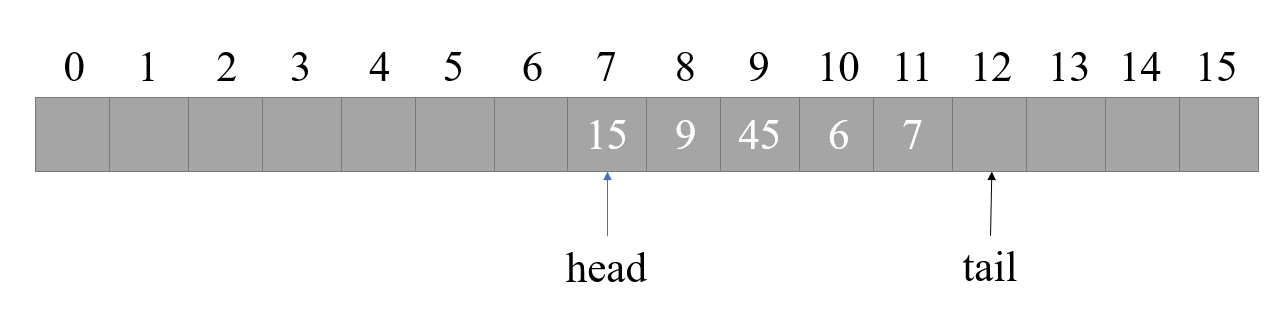// 队列定义
typedef struct Queue{
int* array;
int length;
int tail;
}Queue;


// 初始化
Queue* QueueInit(int queue_length){
Queue* queue = (Queue*)malloc(sizeof(Queue));
queue->array = (int*)malloc(sizeof(int) * (queue_length + 1));
queue->tail = 0;
queue->length = queue_length + 1;
return queue;
}


// 判断队列是否已满
int QueueIsFull(Queue* queue){
return (((queue->tail + 1) % queue->length) == queue->head);
}

// 判断队列是否为空
int QueueIsEmpty(Queue* queue){
}


// 入队
int QueueEnqueue(Queue* queue, int number_to_enqueue){
if(QueueIsFull(queue)){
return -1;
}

queue->array[queue->tail] = number_to_enqueue;
queue->tail  = (queue->tail + 1) % queue->length;
return 0;
}


// 出队
int QueueDequeue(Queue* queue){
return return_value;
}


### 有根树

#### 二叉树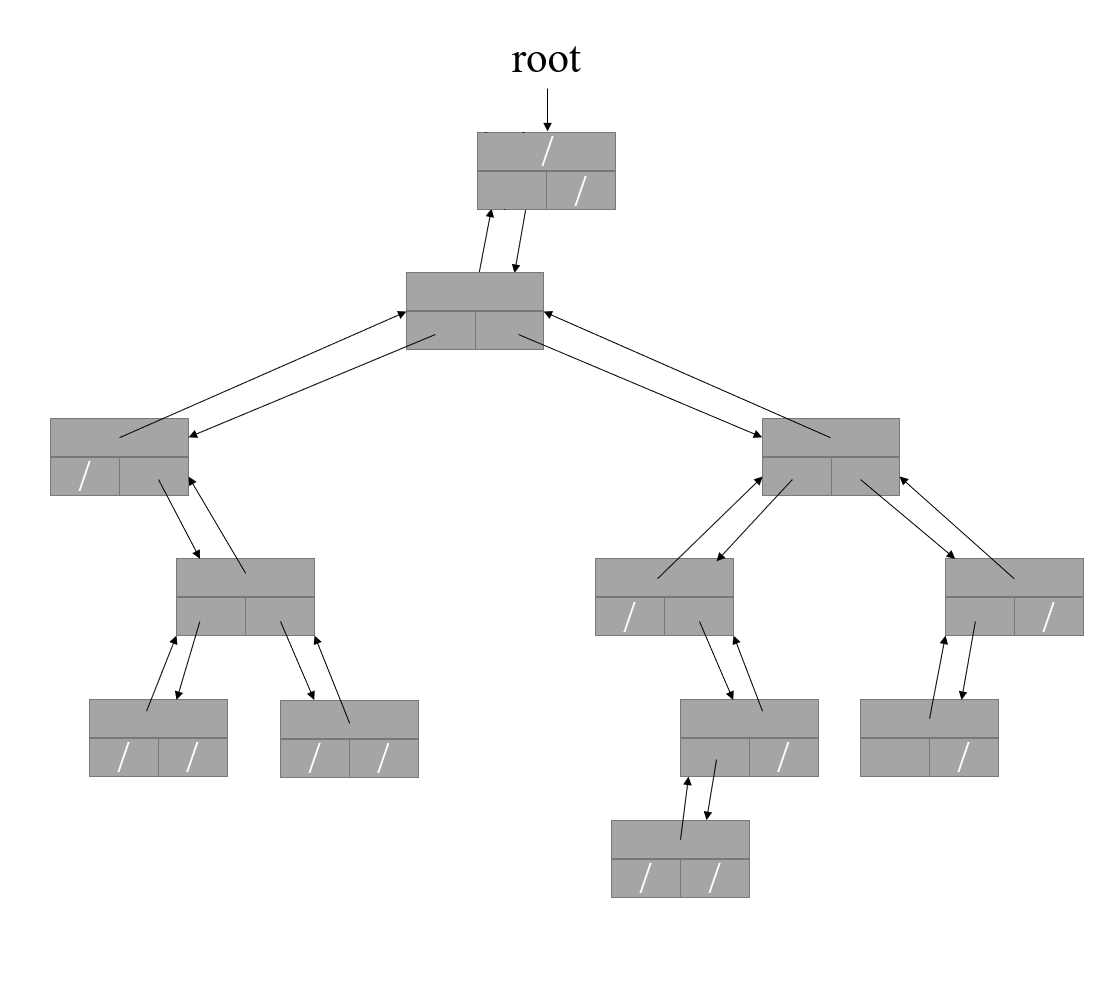#### 分支无限制的有根树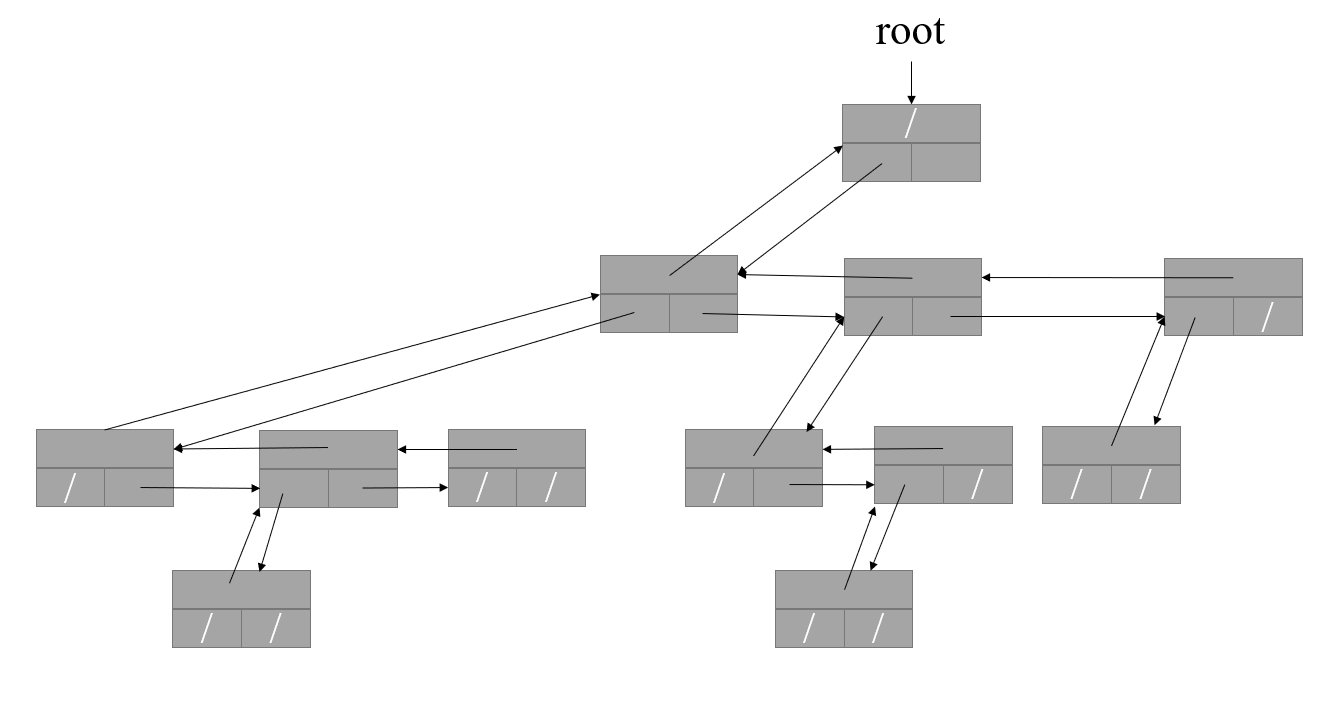0
0 收藏

### 作者的其它热门文章0 评论
0 收藏
0